All You Need to Know About

# SUMPRODUCT Formula in Excel

The Excel SUMPRODUCT function returns the sum of the products of corresponding ranges or arrays. Read more about the SUMPRODUCT Function in Excel below!

Here are the top things on what you can do with the SUMPRODUCT Formula in Excel:

## SUMPRODUCT & Weighted Averages

What does it do?

It returns the sum of the products of corresponding ranges or arrays

Formula breakdown:

=SUMPRODUCT(array1, [array2], [array3]…)

What it means:

=SUMPRODUCT(this array, with that array…)

A quick way to calculate the weighted average of two lists of data is to use the SUMPRODUCT formula.  A weighted average can be used to determine the average salary of employees, the average grade of an exam or the average selling price of a company´s stock list, as can been seen below.

We want to get the average selling price of our total stock items. This is easily achievable with the SUMPRODUCT formula! We will use this to calculate the total value of the items, then divide this by the total number of units to get the average selling price.

STEP 1: We need to enter the SUMPRODUCT function in a blank cell:

## =SUMPRODUCT(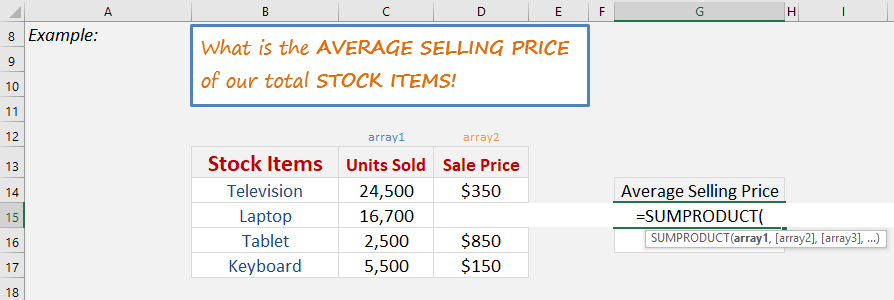STEP 2: The SUMPRODUCT arguments:

## Array1

What is the first array that contains the data?

We want to get the units sold so select those values.

## =SUMPRODUCT(C14:C17,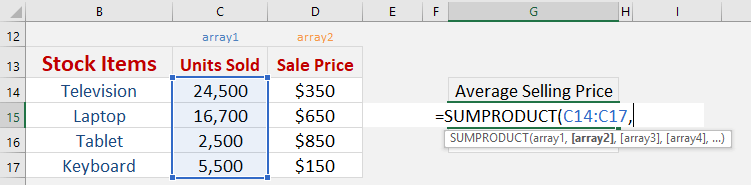## Array2

What is the second array that contains the data?

We want to get the sale price sold so select those values. The values will be multiplied against the first array that we got.

## =SUMPRODUCT(C14:C17, D14:D17)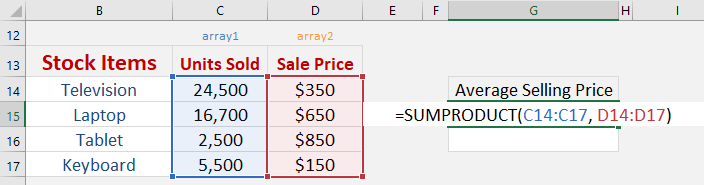STEP 3: Now we have the total value, we can easily get the average value by dividing by the total number of items.

## =SUMPRODUCT(C14:C17, D14:D17) / SUM(C14:C17)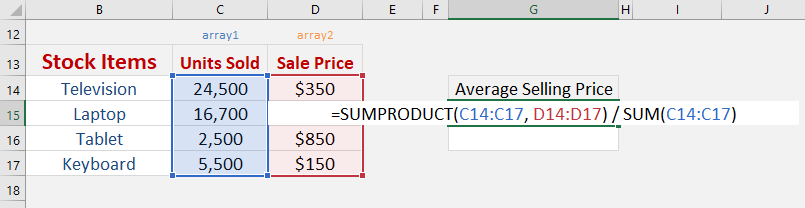With just this single formula, we are able to get the average selling price without the need of extra helper columns!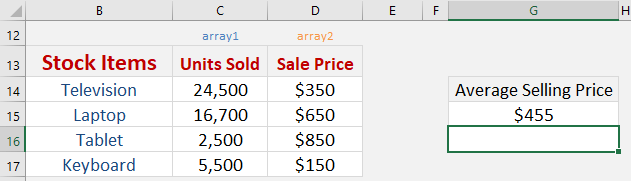## Advanced SUMPRODUCT Function: Conditional Date

If you want to find out the total sales for a particular month, then the SUMPRODUCT function is your answer.  You can create a criteria for a specific date range, a particular month or a year.

In the example below I show you how to use the SUMPRODUCT function to sum up the total sales for a particular month.

## Advanced SUMPRODUCT Function: Maximum Sales

What makes the SUMPRODUCT function even more powerful is its ability to nest formulas, or in simple terms, add another function within the SUMPRODUCT function.

Instead of getting the Total Sales for a region, we can extract the Maximum sales value simply by entering the MAX function before the criteria.  See how below.

Latest Tutorials

June 19, 2020

## Advanced Sumproduct Function: Sum the Top 3 Sales with an Array Formula

September 23, 2014

## Advanced SUMPRODUCT Function: Maximum Sales

September 23, 2014

## Advanced SUMPRODUCT Function: Conditional Date

September 23, 2014

## Advanced SUMPRODUCT Function: Conditional Sum

September 23, 2014